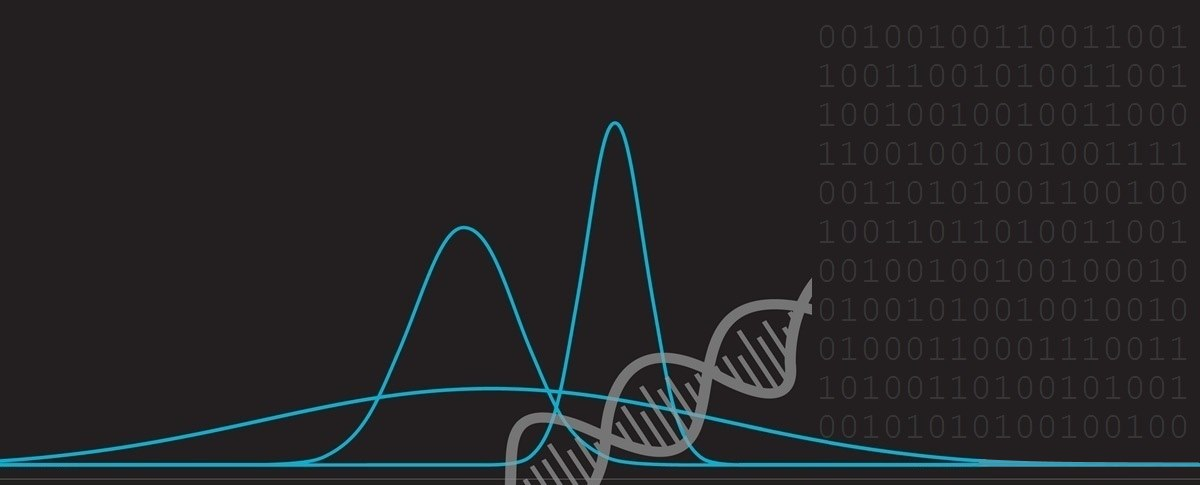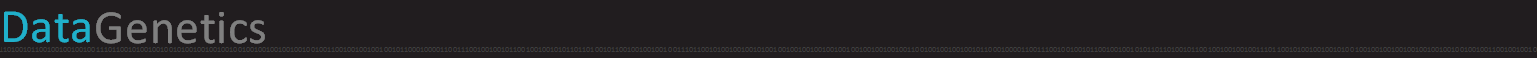# Counterintuitive Conundrums

In this posting we’re going a have fun examining a few counterintuitive puzzles. Now, even though I’ve already tipped my hand, and you know that each answer is probably not going to be the first thing that comes into your mind, spend a few minutes thinking about each puzzle before reading the solution.I promise that none of the puzzles involve tricks or groan-inducing traps – however they are questions that, when you hear the answer, I suspect that some of you will be surprised to the point of disbelief. Finally, we’ll walk through the surprising solution to each problem explaining the mechanism and proof. I promise, none of the questions are the proverbial Monte-Hall problem. Here we go …

### 1. Band around the World

Imagine that you equipped the Earth with tightly fitting steel belt running all around the equator (assume, for this puzzle, that the Earth is a perfect sphere). The belt fights taut and snug.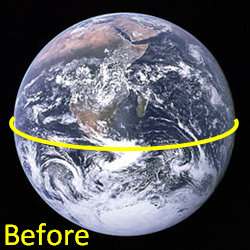Now, imagine you took a saw, cut the belt, added an extra 2 meter section of belt, and welded it back together. The slightly-longer belt will now be a little bit loose, and stand proud of the surface of the Earth all the way around. [For those not familiar with the Metric system, 2 meters is just over six and half feet.]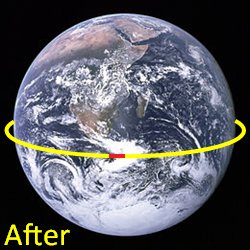Question: How big will the gap between the planet and the band be?

Will the gap be big enough for a couple of air-molecules to pass under? Big enough to slide a playing card under? Big enough to slide your hand under? How about a telephone directory? Would it be big enough for a cat to crawl under? How about a person?

### 2. Giant Jelly

 Imagine you have giant jelly. The jelly weighs 100 pounds, and is 99% water by weight. You leave the jelly out, and let water evaporate from the jelly until it reaches 98% water by weight. Question: How much does the jelly now weigh?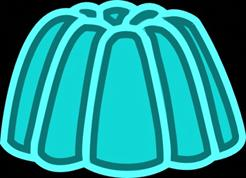### 3. Second Black Ace

 Someone hands you a deck of cards which you thoroughly shuffle. Next, you start to deal them, face-up, counting the cards as you go. “One, Two, Three …” The aim is to predict what the count will be when you encounter the second black Ace in the deck. Question: If you had to select one position before you started to deal, what number would you select that maximizes your chance of guessing the location of the second black Ace?### 4. Aces in Bridge Hands

 Someone deals you a bridge hand (13 cards from a regular deck of 52 cards). You look at the hand and notice you have an Ace and say “I have an Ace”. What is the probability that you have another Ace? The cards are collected and different hand is dealt. This time you look at your hand and state “I have the Ace of Spades” (which is true), what is the probability, this time, that you have another Ace? Question: Is the probability in the second case the same as before, a lower probability, or a higher probability?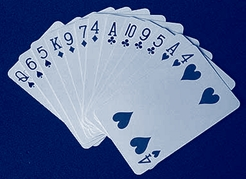No peeking! Did you think about them first?

### 1. Band around the World (Answer)

The surprising answer is that the gap would be big enough to crawl under!

That’s right, the band would stand just over 31.83 cm away of the surface all the way around. That’s over one foot! Certainly enough to crawl under.

We can see the math below. The gap on each side of the planet is 1/Π meters.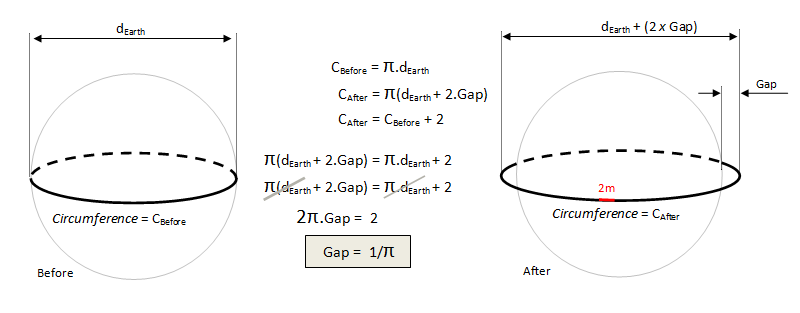Interestingly, the answer is independent of the diameter of the Earth!!

The same size gap would appear if band were placed around the Moon and expanded, or Mars, or the Sun, or even a football or an orange! It's always a 1/Π gap when 2 meters is added!

 The surprising answer is that the jelly would now weigh 50 pounds! When the jelly weighs 100 pounds, it is 99% water. That means that it is 1% non-water, and this non-water component of the jelly must weigh one pound. After evaporation, the non-water component accounts for 2% of the weight of the jelly. So 2% of the jelly weighs one pound. Scaling this up, we see that, if 2% weighs one pound, then the entire jelly must weigh fifty pounds.### 3. Second Black Ace (Answer)

The surprising answer is that you should choose position 52 (the last card!)

Whilst there is a lot of math that can be used to prove this, it’s probably easier to simply think of it this way: It’s random where the two black aces are in the deck, but only the last position of the deck can you guarantee that it will be the second Ace (any other position you find a black Ace, there is a chance it could be the first black Ace).

Similarly, if you had to select a count to maximize your chances of finding the first black Ace you should select the first card. Only the first card can you guarantee, if it is a black Ace, that it is the first black Ace. (All other positions, if there is a black Ace, there is a chance that there is another black Ace infront of it).

For those interested in a more general solution, instead of black Aces, let’s imagine we had to guess the most likely position of every Ace. As before, the first and last positions are the ones we should select for the first and fourth Ace, but to calculate the most likely position for the second and third Ace, we need a little math.

To calculate the probability of the second ace occurring in position n, we can do something like this:

Since this will be the second Ace, there needs to be another Ace in the first (n-1) cards.

Each selection of (n-1) cards has the probability of (53-n)! / 52!, and of these the number that contain just one Ace is (n-1) x 4 x 48! / (50-n)!

Finally, the nth card must also be an Ace, and this occurs with a probability of 3 / (53-n)

All we need to do now is multiply these probabilities together (the logical way of AND), and this will give us the probability of the second Ace appearing in position n in the deck.

= ((53-n)! / 52!) x ((n-1) x 4 x 48! / (50-n)!) x (3 / (53-n))

= 12 x (n-1) x ((48! / 52!) x ((52-n)! / (50-n)!)

= 12 x (48! / 52!) x (n-1) x (52-n) x (51-n)

= (12 / (52 x 51 x 50 x 49)) x (n-1) x (52-n) x (51-n)

We could break out some Calculus to caclulate and solve the maximum of this equation for n, but let's go old school and graph it out …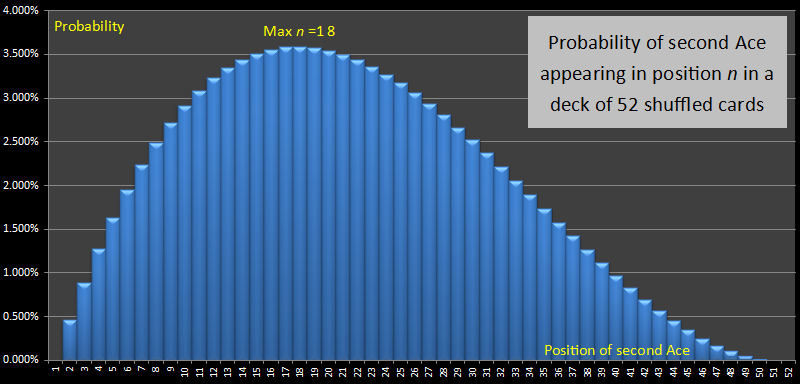The cubic function has a maximum at n=18, and so the most likely position for the second of four Aces is position 18. A similar calculation for the third Ace shows that the most likely position for this is position 35.

So the general solution – the most likely positions for the 1st, 2nd, 3rd, 4th Aces in a deck are #1, #18, #35, #52.

### 4. Aces in Bridge Hands (Answer)

 The surprising answer is that, if you call “I have the Ace of Spades”, it is more likely that you have another Ace than if you just state “I have an Ace”! If you state you have the Ace of Spades there is an 11686 / 20825 chance you have (at least) another Ace, which is 56.12%. If you state you have An Ace then the chance you have (at least) another Ace is 5359 / 14498 (which is 36.27%). It’s over 50% less likely! How come? Well, this puzzle is more about a confidence problem than a probability problem. It’s about the disclosure of information.Thinking about it another way. What this puzzle is really about is comparing the probabilities of:

 # hands with at least two Aces / # hands with at least one Ace and # hands with at least two Aces (one of which is the Ace of Spades) / # hands with the Ace of Spades

OK, hold onto your hats, here we go with the math. To keep the equations of manageable size, I’m going to use the abbreviation b{n,r} to represent the binomial expansion function n!/(r!(n-r)!) (often referred to as n choose r).

First a few basics:

Total_Number_Hands — All possible ways to combine the cards to make a Bridge hand. There are 52 cards in a deck, and 13 cards in a hand = b{52,13}

No_Aces — The number of hands that have no Aces. There are 48 non-Ace cards, and 13 in a hand = b{48,13}

At_Least_One_Ace — The number of hands that have at least one Ace = Total_Number_Hands - No_Aces

Excatly_One_Ace — The number of hands that have just one Ace. (Chose any Ace, then choose 12 non-aces) = b{4,1} x b{48,12}

At_Least_Two_Aces — Start with the total number of hands, then subtract hands with either no Aces, or one Ace. You are left with the number of hands with two or more Aces = Total_Number_Hands - (No_Aces + Exactly_One_Ace)

We now have enough to calculate our first ratio (chances of having a second Ace if you state you have an Ace):

At_Least_Two_Aces / At_Least_One_Ace = 36.27%

To calculate the ratio where the person claims to have the Ace of Spades, we need some additional terms:

With_Ace_Spades — Number of hands with Ace of Spades. (We choose the Ace of Spades, and then have to choose an additional 12 cards from the remaining 51) = b{51,12}

No_Other_Aces — Number of hands with no other Aces = b{48,12}

At_Least_Two_Aces_(Including_Ace_Spade) — Number of hands with at least two Aces, one of which is the Ace of Spades = With_Ace_Spades - No_Other_Aces

We now have enough to calculate our second ratio (chances of having a second Ace if you state you have the Ace of Spades):

There you have it!

### The second child (bonus!)

A similar paradox is the Paradox of the second Child. If you meet someone and they tell you “I have two children, and at least one of them is a boy”, what is the probability that the other child is a boy? (Hint: It’s not 1/2). It’s actually 1/3.

However if the person says “I have two children and the eldest is a boy” (or the tallest, or the neatest, or the heaviest … ) then the probability that the other child is a boy is indeed 1/2. How does the act of not specifying reduce the probabilities?Again, it’s more a concept of disclosure of information. There are four combinations for the gender of two children: MM, MF, FM, FF. If the first case, “at least one is a boy”, there are three possible, equally likely, cases: MM, MF, FM. (The only case it could not be is FF). There is, thus, only one chance in three that the other child is also a boy. When you specify which child it is (let’s call it the first child), your solution set reduces to: MM, MF and so the chance that the other child is a boy is the logical 50:50 chance you’d expect.

Not convinced? Try flipping two same denomination coins, and using heads and tails to represent boys and girls. If you flip two tails, ignore it (both girls), but if at least one of them is a head, then count the number of times the other coin is also a head. Note the results after a few dozen flips.

 Next repeat the experiment with two different denomination coins. One representing the eldest child, and the other the youngest. When the eldest lands on a head, count the number of times the other coin is also a head. Get it now?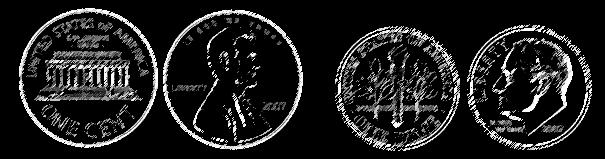# Martin Gardner 1914-2010Many of these puzzles were inspired, or even copied directly, from the creations of Martin Gardner. I read my first compendium of his work when I was 17 years old, and went on to consume and enjoy everything he produced. He was a true inspiration to me. If you love math, or science, or puzzles, or magic – read his work. “He turned thousands of children into mathematicians, and thousands of mathematicians into children.” – John Tierney.

You can find a complete list of all the articles here.Click here to receive email alerts on new articles.